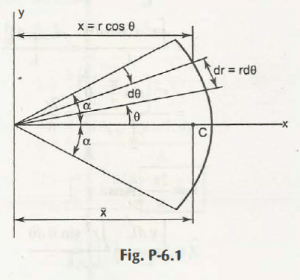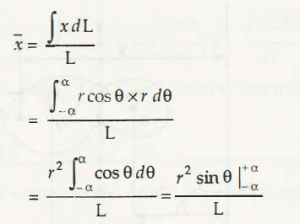Centre of mass of an object is the point where the weight or mass is considered as zero. In the words, the point of relative mass of the object is the position from where the mass is considered to be distributed to the entire object.

For a simple rigid body whose density is uniformly distributed throughout the area has its centre of mass at its centroid. It also happens that centre of mass of an object falls outside the cross-sectional area of an object.

The above explanation specifies that centre of mass can be located at any point either inside the body or outside the body depending upon its mass density.

Coordinate of centre of mass:

Let us consider a composite body is divided into small elements as shown in figure below-